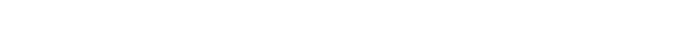Published on

# Definition of Base64

Base64 is a encoding scheme used to represent arbitrary data with US-ASCII-compatible strings. The alphabet of an encoded string consists of `2^6 + 1 = 65` characters, where the first `64` characters represent the actual values and the last one (`=`) is used for padding when needed. Each of the `2^6 = 64` value characters represents `6` bits of the data.

See below for a listing of the full alphabet:

Character Value (bin) Value (hex) Value (dec)
`A` `000000` `00` `0`
`B` `000001` `01` `1`
`C` `000010` `02` `2`
`D` `000011` `03` `3`
`E` `000100` `04` `4`
`F` `000101` `05` `5`
`G` `000110` `06` `6`
`H` `000111` `07` `7`
`I` `001000` `08` `8`
`J` `001001` `09` `9`
`K` `001010` `0A` `10`
`L` `001011` `0B` `11`
`M` `001100` `0C` `12`
`N` `001101` `0D` `13`
`O` `001110` `0E` `14`
`P` `001111` `0F` `15`
`Q` `010000` `10` `16`
`R` `010001` `11` `17`
`S` `010010` `12` `18`
`T` `010011` `13` `19`
`U` `010100` `14` `20`
`V` `010101` `15` `21`
`W` `010110` `16` `22`
`X` `010111` `17` `23`
`Y` `011000` `18` `24`
`Z` `011001` `19` `25`
`a` `011010` `1A` `26`
`b` `011011` `1B` `27`
`c` `011100` `1C` `28`
`d` `011101` `1D` `29`
`e` `011110` `1E` `30`
`f` `011111` `1F` `31`
`g` `100000` `20` `32`
`h` `100001` `21` `33`
`i` `100010` `22` `34`
`j` `100011` `23` `35`
`k` `100100` `24` `36`
`l` `100101` `25` `37`
`m` `100110` `26` `38`
`n` `100111` `27` `39`
`o` `101000` `28` `40`
`p` `101001` `29` `41`
`q` `101010` `2A` `42`
`r` `101011` `2B` `43`
`s` `101100` `2C` `44`
`t` `101101` `2D` `45`
`u` `101110` `2E` `46`
`v` `101111` `2F` `47`
`w` `110000` `30` `48`
`x` `110001` `31` `49`
`y` `110010` `32` `50`
`z` `110011` `33` `51`
`0` `110100` `34` `52`
`1` `110101` `35` `53`
`2` `110110` `36` `54`
`3` `110111` `37` `55`
`4` `111000` `38` `56`
`5` `111001` `39` `57`
`6` `111010` `3A` `58`
`7` `111011` `3B` `59`
`8` `111100` `3C` `60`
`9` `111101` `3D` `61`
`+` `111110` `3E` `62`
`/` `111111` `3F` `63`

Encoding `24` bits (`3` bytes) of data, takes `4` characters in Base64 (`4` * `6` bits = `24` bits). If the data is not a multiple of `3` bytes, we have to append zero-bytes (i.e. `00000000`) until it is (at most we have to append `2` such bytes). Afterwards, we split the data into `3`-byte chunks, to get a sequence of so-called quanta and proceed by encoding each quantum as follows.

Encoding some data with up to `3` bytes looks like this:

Data (# bytes) + zero-bytes Base64 with padding
(0)
`00000100` (1) + `0000000000000000` `BAAA` `BA==`
`0000010000010000` (2) + `00000000` `BBAA` `BBA=`
`000001000001000001000001` (3) `BBBB` `BBBB`

The number of characters at the end of the Base64 string to replace with the padding character (`=`) can be calculated as follows for a data with `n` bytes:The number of required value bits `v` and padding bits `p` are easy to calculate for a given number of data bits `n`:Note, that `24` is the smallest common multiple of `6` and `8`.

## Observations

• Base64 strings are invalid (i.e., cannot be decoded) if they contain any characters outside the alphabet given above.
• Due to the padding, the length (number of characters) in valid Base64 strings is always divisible by `4`. Therefore, Base64 strings are also invalid, if this is not the case.
• Base64 is of course fully reversible: `decode64(encode64(d)) = d` for some arbitrary data `d` with `n >= 0` bytes. Therefore, libraries usually provide an `encode` and a `decode` function.